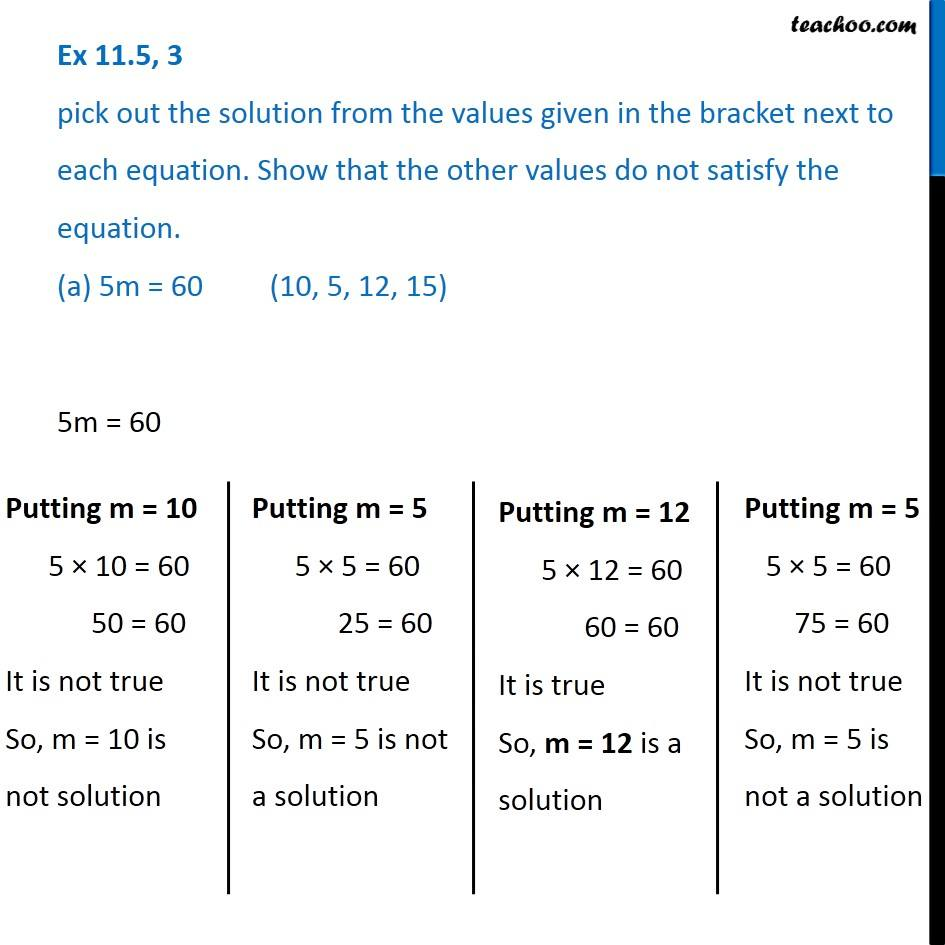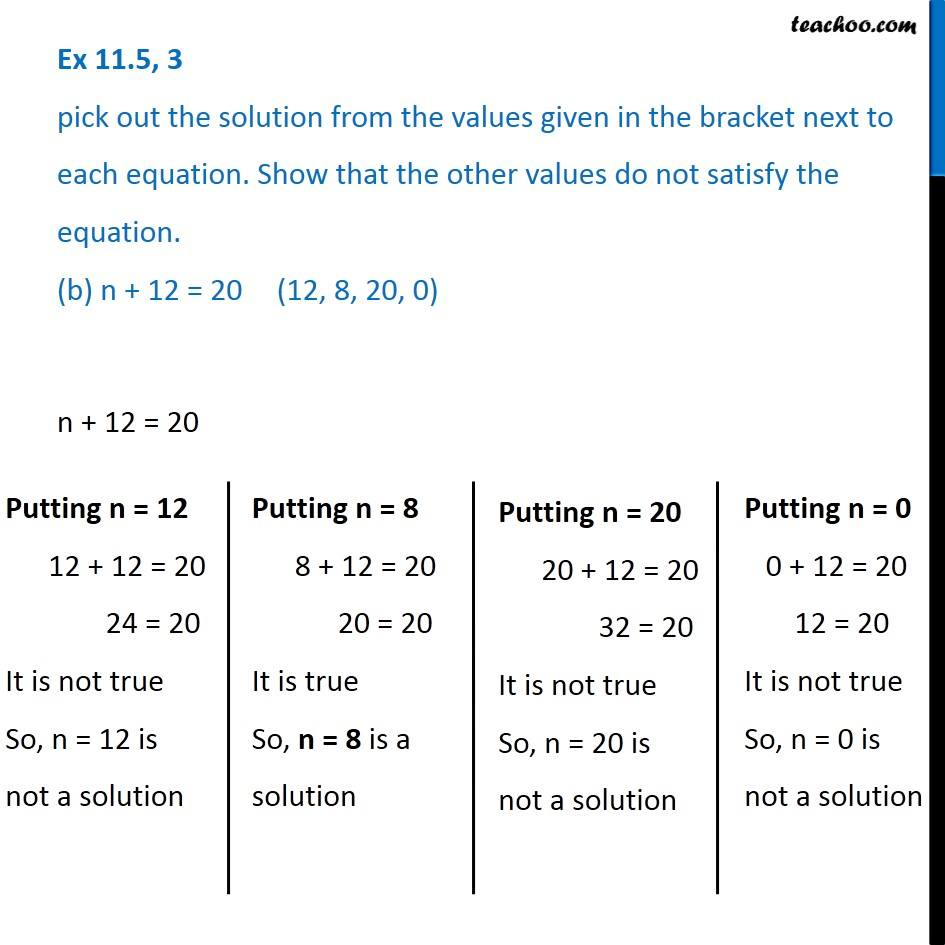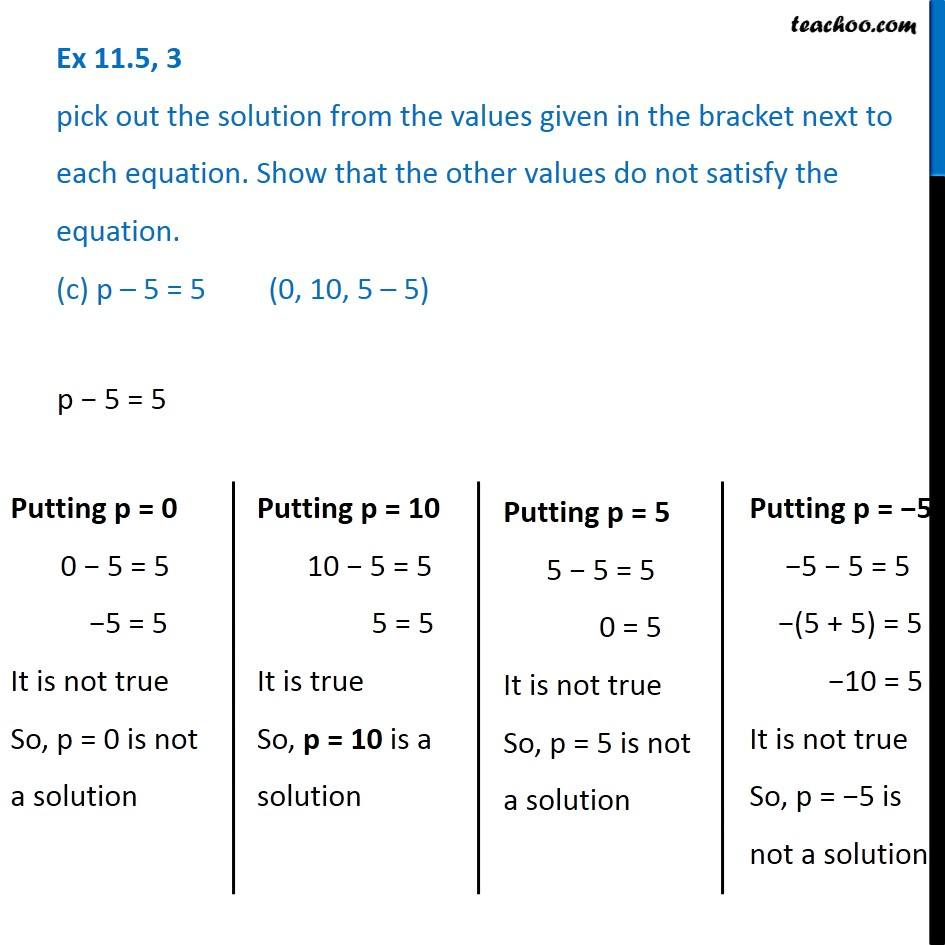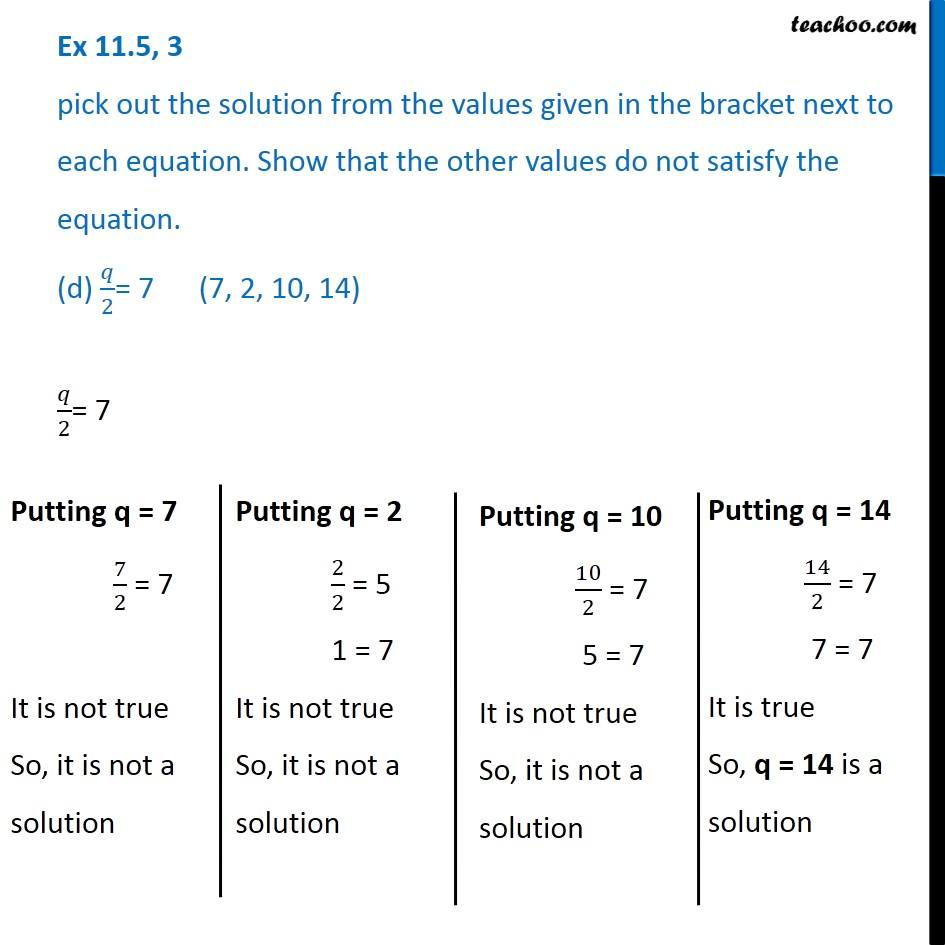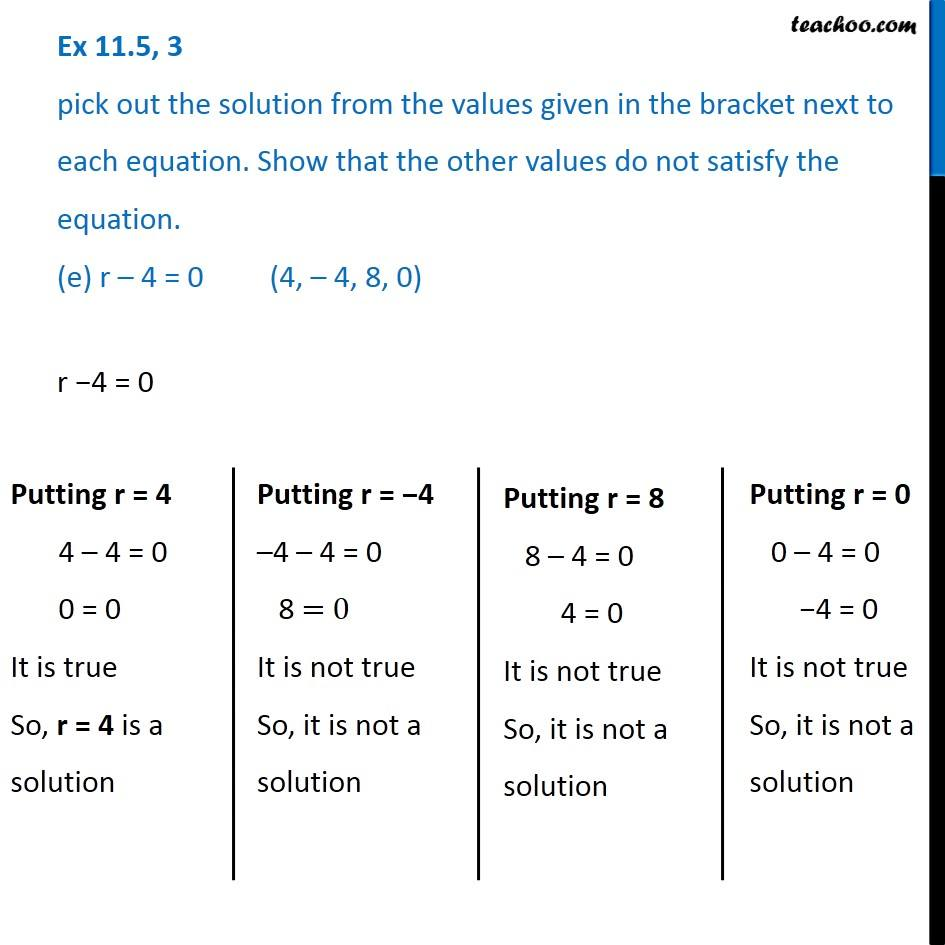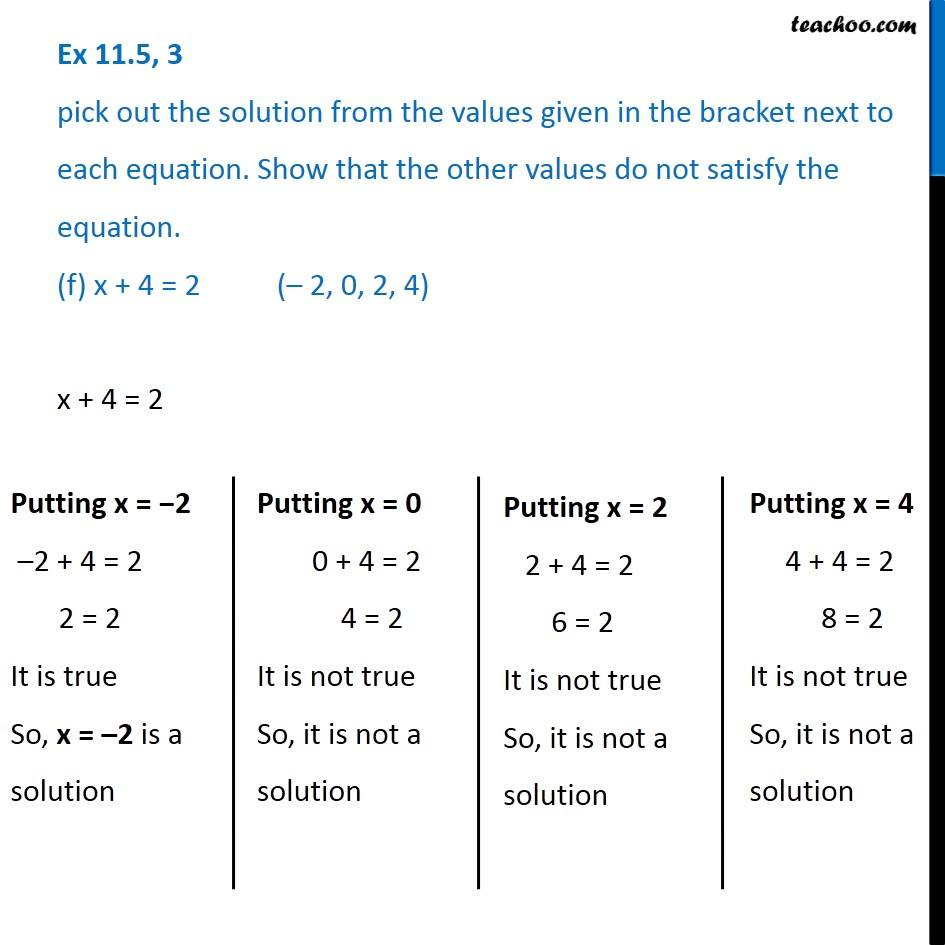Subscribe to our Youtube Channel - https://you.tube/teachoo

1. Chapter 11 Class 6 Algebra
2. Serial order wise
3. Ex 11.5

Transcript

Ex 11.5, 3 pick out the solution from the values given in the bracket next to each equation. Show that the other values do not satisfy the equation. (a) 5m = 60 (10, 5, 12, 15) 5m = 60 Putting m = 10 5 × 10 = 60 50 = 60 It is not true So, m = 10 is not solution Putting m = 5 5 × 5 = 60 25 = 60 It is not true So, m = 5 is not a solution Putting m = 12 5 × 12 = 60 60 = 60 It is true So, m = 12 is a solution Putting m = 5 5 × 5 = 60 75 = 60 It is not true So, m = 5 is not a solution Ex 11.5, 3 pick out the solution from the values given in the bracket next to each equation. Show that the other values do not satisfy the equation. (b) n + 12 = 20 (12, 8, 20, 0)n + 12 = 20 Putting n = 12 12 + 12 = 20 24 = 20 It is not true So, n = 12 is not a solution Putting n = 8 8 + 12 = 20 20 = 20 It is true So, n = 8 is a solution Putting n = 20 20 + 12 = 20 32 = 20 It is not true So, n = 20 is not a solution Putting n = 0 0 + 12 = 20 12 = 20 It is not true So, n = 0 is not a solution Ex 11.5, 3 pick out the solution from the values given in the bracket next to each equation. Show that the other values do not satisfy the equation. (c) p – 5 = 5 (0, 10, 5 – 5)p − 5 = 5 Putting p = 0 0 − 5 = 5 −5 = 5 It is not true So, p = 0 is not a solution Putting p = 10 10 − 5 = 5 5 = 5 It is true So, p = 10 is a solution Putting p = 5 5 − 5 = 5 0 = 5 It is not true So, p = 5 is not a solution Putting p = −5 −5 − 5 = 5 −(5 + 5) = 5 −10 = 5 It is not true So, p = −5 is not a solution Ex 11.5, 3 pick out the solution from the values given in the bracket next to each equation. Show that the other values do not satisfy the equation. (d) 𝑞/2= 7 (7, 2, 10, 14) 𝑞/2= 7 Putting q = 7 7/2 = 7 It is not true So, it is not a solution Putting q = 2 2/2 = 5 1 = 7 It is not true So, it is not a solution Putting q = 2 2/2 = 5 1 = 7 It is not true So, it is not a solution Putting q = 10 10/2 = 7 5 = 7 It is not true So, it is not a solution Putting q = 10 10/2 = 7 5 = 7 It is not true So, it is not a solution Putting q = 14 14/2 = 7 7 = 7 It is true So, q = 14 is a solution Ex 11.5, 3 pick out the solution from the values given in the bracket next to each equation. Show that the other values do not satisfy the equation. (e) r – 4 = 0 (4, – 4, 8, 0)r −4 = 0 Putting r = 4 4 – 4 = 0 0 = 0 It is true So, r = 4 is a solution Putting r = −4 –4 – 4 = 0 8 = 0 It is not true So, it is not a solution Putting r = 8 8 – 4 = 0 4 = 0 It is not true So, it is not a solution Putting r = 0 0 – 4 = 0 −4 = 0 It is not true So, it is not a solution Ex 11.5, 3 pick out the solution from the values given in the bracket next to each equation. Show that the other values do not satisfy the equation. (f) x + 4 = 2 (– 2, 0, 2, 4)x + 4 = 2 Putting x = −2 –2 + 4 = 2 2 = 2 It is true So, x = –2 is a solution Putting x = 0 0 + 4 = 2 4 = 2 It is not true So, it is not a solution Putting x = 2 2 + 4 = 2 6 = 2 It is not true So, it is not a solution Putting x = 2 2 + 4 = 2 6 = 2 It is not true So, it is not a solution Putting x = 2 2 + 4 = 2 6 = 2 It is not true So, it is not a solution Putting x = 4 4 + 4 = 2 8 = 2 It is not true So, it is not a solution

Ex 11.5

Chapter 11 Class 6 Algebra
Serial order wise